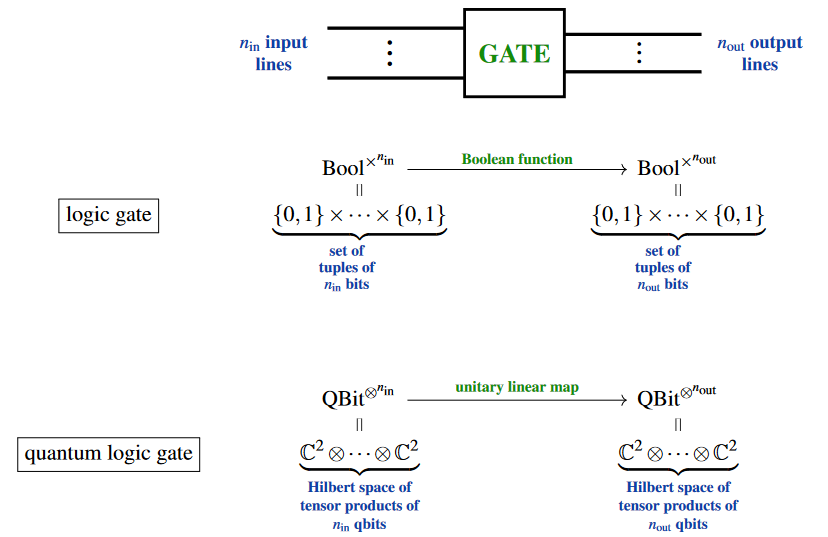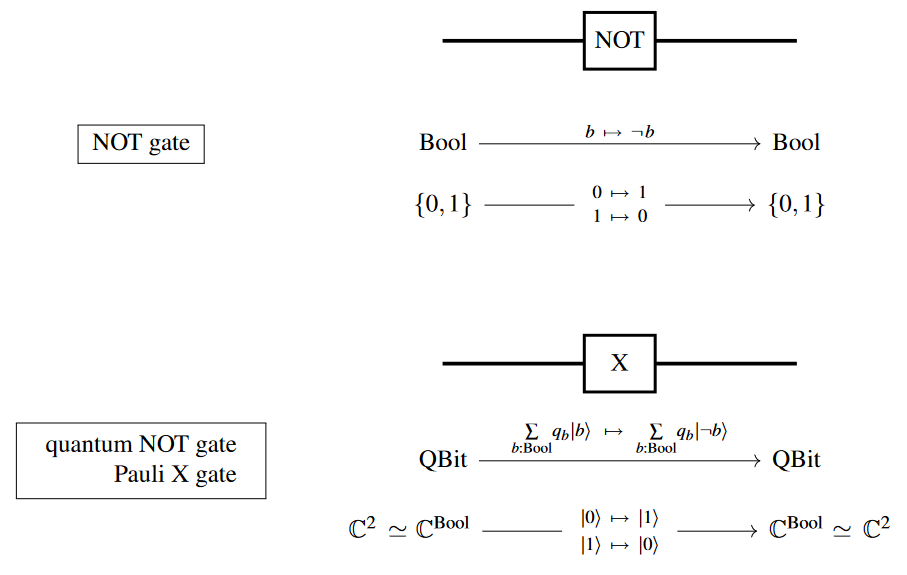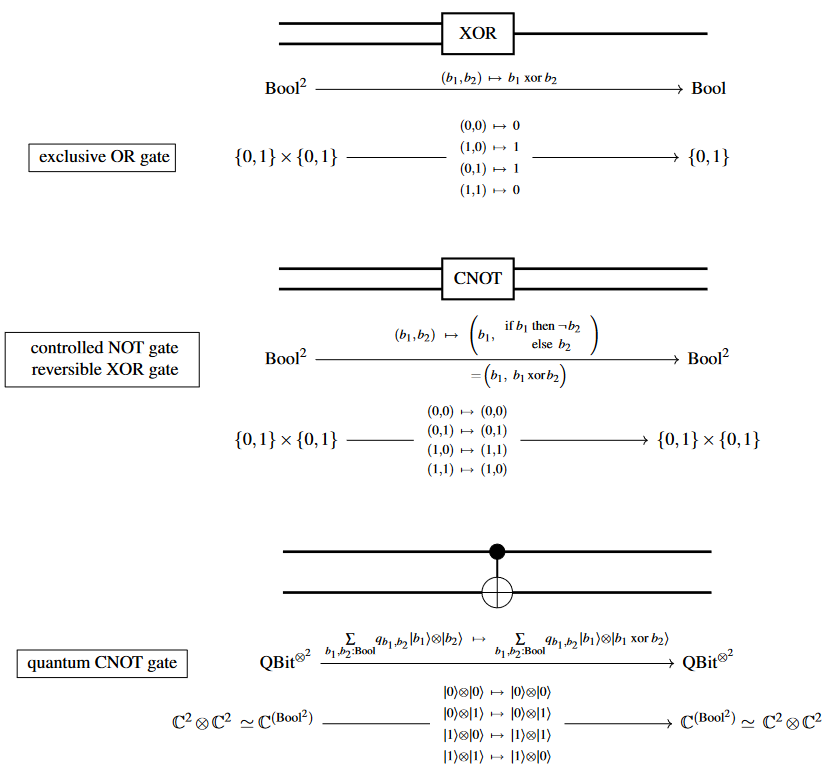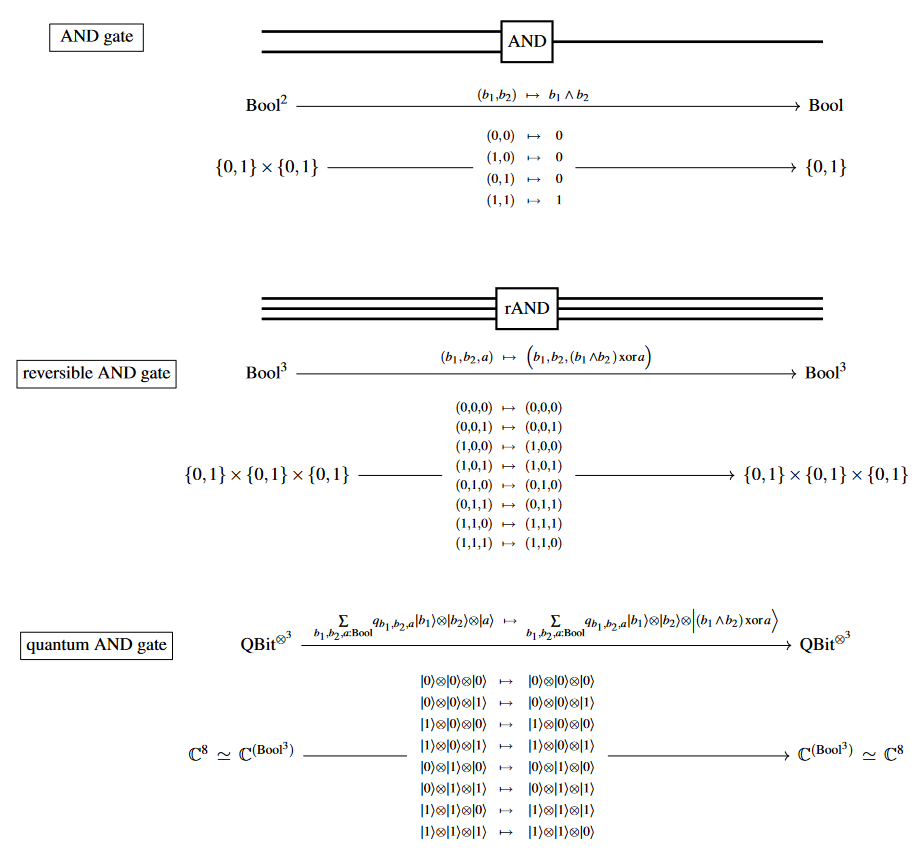# nLab logic gate

Contents

### Context

#### Computation

intuitionistic mathematics

# Contents

## Idea

Broadly, a logic gate is a function of tuples of truth values, hence a map between cartesian products $\mathrm{Bool}^n$ of Booleans $Bool \,\coloneqq\, \{false, true\} \;\simeq\; \{0,1\}$.Specifically, one calls such a function a logic gate if it is thought of as potentially implemented as a basic operation performed by a computing machine – in which case the truth values are also called bits.

As such, typical logic gates are Boolean functions between a small number of bits, with more complicated such functions thought as obtained by composing a given set of logic gates into logic circuits.

Analogously there are quantum logic gates compiled into quantum circuits.

## Examples

A list of some basic logic and gates and their reversible and quantum logic gate versions:

NOT:XOR and CNOT:AND:(…)

Textbook accounts:

• Jan Friso Groote, Rolf Morel, Julien Schmaltz, Adam Watkins, Logic Gates, Circuits, Processors, Compilers and Computers, Springer (2021) [doi:10.1007/978-3-030-68553-9]

• John Bird, Chapter 7 of: Bird’s Higher Engineering Mathematics, Routledge (2021) [ISBN:9781003124221]